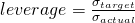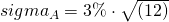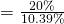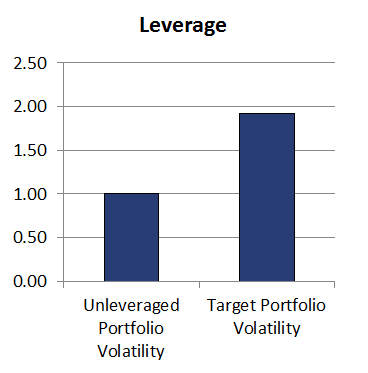## Volatility targeting

Volatility targeting is a sophisticated approach used by hedge funds to determine the amount of leverage that will be used. Volatility targeting is strongly related to trend following, since its most commonly used by commodity trading advisors (CTAs).

It is a form of risk management applied at the level of the portfolio. The approach is closely related to equal volatility weighting and risk parity. On this page, we provide a simple definition of volatility targeting, give an example of volatility targeting, and discuss some of the advantages and disadvantages of using volatility targeting.

## Volatility targeting definition

Volatility targeting means that the manager will increase or decrease the amount of leverage to ensure that the volatility of the portfolio remains close to the target. As such, if the volatility goes up, he or she will scale down the portfolio. If the volatility goes down, the manager will take more leverage. The aim is to ensure that the amount of dollar risk remains the same.

To perform volatility targeting, two elements are needed.

• The first one is the volatility target. This is the level of annual volatility to which we want to adjust the portfolio. We will adjust the portfolio volatility by lowering or increasing the amount of leverage
• The second element is the actual annualized volatility of the portfolio. This is the level of volatility of the unleveraged or unlevered portfolio.

Mathematically, the amount over leverage equals:## Example volatility targeting

To make this clearly, let’s consider a numerical example. Suppose we have a number of securities. We apply a particular weighting scheme and we find that the portfolio volatility of this unlevered portfolio is 3% per month. First, we determine the annualized volatilityOr 10.39% p.a. Suppose this level of volatility is too low. Let’s imagine we want this portfolio to trade at 20% volatility per year. In that case, we need to apply the following amount of leverageOr 1.92. This means that, for every dollar we invest, we need to borrow an additional \$0.92 to ensure that the portfolio achieves the target volatility of 20% per year. The target volatility depends on the amount of risk that the investor is willing to take. The more volatile the portfolio and the higher the amount of leverage used, the riskier the portfolio.## Summary

We discussed a risk management technique called volatility targeting. It is often used by CTAs to ensure that the portfolio exhibits the level of volatility that is needed. The manager will periodically adjust the amount of leverage to ensure that the portfolio meets the level of target volatility.